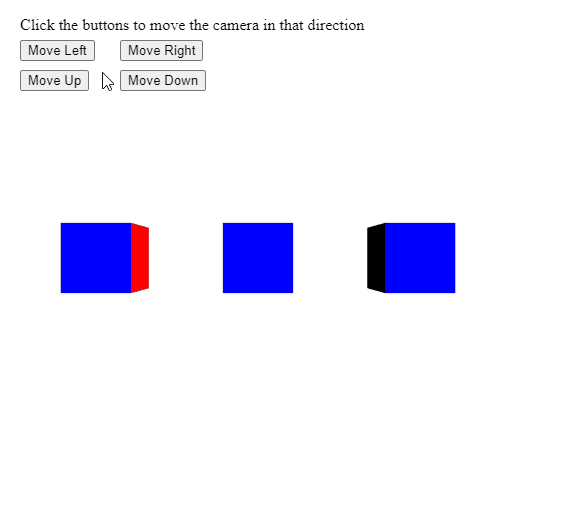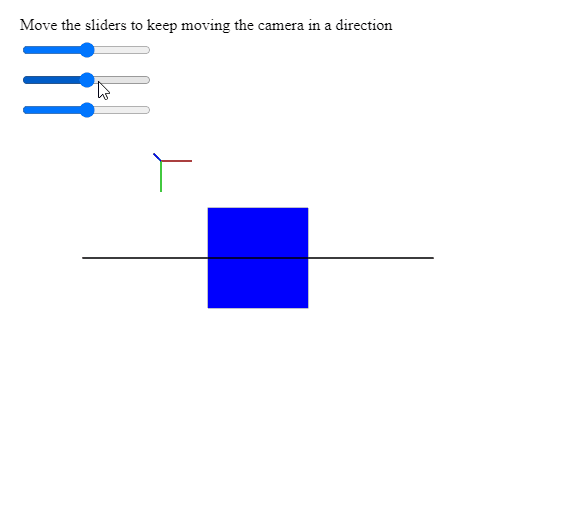GeeksforGeeks App
Open AppBrowser
Continue

## Related Articles

The move() method of p5.Camera in p5.js is used to move the camera along its local axes by the specified amount. It maintains the current camera orientation while moving.

Syntax:

`move( x, y, z )`

Parameters: This method accepts three parameters as mentioned above and described below:

• x: It is a number that denotes the amount to move the camera along its left-right axis.
• y: It is a number that denotes the amount to move the camera along its up-down axis.
• z: It is a number that denotes the amount to move the camera along its forward-backward axis.

The example below illustrates the move() method in p5.js:

Example 1:

## Javascript

 `let currCamera;`` ` `function` `setup() {``  ``createCanvas(500, 500, WEBGL);``  ``helpText = createP(``    ``"Click the buttons to move the "` `+``    ``"camera in that direction"``);``  ``helpText.position(20, 0);`` ` `  ``currCamera = createCamera();`` ` `  ``// Create three buttons for moving the``  ``// position camera``  ``newCameraBtn = createButton(``"Move Left"``);``  ``newCameraBtn.position(20, 40);``  ``newCameraBtn.mouseClicked(moveCameraLeft);``   ` `  ``newCameraBtn = createButton(``"Move Right"``);``  ``newCameraBtn.position(120, 40);``  ``newCameraBtn.mouseClicked(moveCameraRight);`` ` `  ``newCameraBtn = createButton(``"Move Up"``);``  ``newCameraBtn.position(20, 70);``  ``newCameraBtn.mouseClicked(moveCameraUp);`` ` `  ``newCameraBtn = createButton(``"Move Down"``);``  ``newCameraBtn.position(120, 70);``  ``newCameraBtn.mouseClicked(moveCameraDown);``}`` ` `function` `moveCameraLeft() {`` ` `  ``// Look at the given position``  ``// in the world space``  ``currCamera.move(-15, 0, 0);``}`` ` `function` `moveCameraRight() {`` ` `  ``// Look at the given position``  ``// in the world space``  ``currCamera.move(15, 0, 0);``}`` ` `function` `moveCameraUp() {`` ` `  ``// Look at the given position``  ``// in the world space``  ``currCamera.move(0, -15, 0);``}`` ` `function` `moveCameraDown() {`` ` `  ``// Look at the given position``  ``// in the world space``  ``currCamera.move(0, 15, 0);``}`` ` `function` `draw() {``  ``clear();``  ``normalMaterial();`` ` `  ``// Create three boxes at three positions``  ``translate(-150, 0);``  ``box(65);``  ``translate(150, 0);``  ``box(65);``  ``translate(150, 0);``  ``box(65);``}`

Output:Example 2:

## Javascript

 `let currCamera;`` ` `function` `setup() {``  ``createCanvas(500, 500, WEBGL);``  ``helpText = createP(``    ``"Move the sliders to keep moving "` `+``    ``"the camera in a direction"``  ``);``  ``helpText.position(20, 0);`` ` `  ``// Create the camera``  ``currCamera = createCamera();`` ` `  ``// Create three sliders for moving the``  ``// position of the camera``  ``xPosSlider = createSlider(-2, 2, 0);``  ``xPosSlider.position(20, 40);`` ` `  ``yPosSlider = createSlider(-2, 2, 0);``  ``yPosSlider.position(20, 70);`` ` `  ``zPosSlider = createSlider(-2, 2, 0);``  ``zPosSlider.position(20, 100);``}`` ` `function` `draw() {``  ``clear();``  ``lights();``  ``normalMaterial();``  ``debugMode();`` ` `  ``// Get the x, y, z values from the``  ``// sliders``  ``let currX = xPosSlider.value();``  ``let currY = yPosSlider.value();``  ``let currZ = zPosSlider.value();`` ` `  ``// Keep moving the camera according to``  ``// to the given amount``  ``currCamera.move(currX, currY, currZ);`` ` `  ``box(90);``}`

Output:Online editor: https://editor.p5js.org/

My Personal Notes arrow_drop_up
Related Tutorials